# Tic Tac Toe CNN

by Daniel Pollithy## Experiment: Writing a CNN for tic tac toe

Let’s write a CNN which detects the current state of a tic tac toe game.

Although it might be super easy to write an opencv2 script which makes this I want to see whether I have learned something from my past book lectures.

## Planning

First of all I am going to write a simple script which generates pixel maps for given tic tac toe states. There are three mutual exclusive states for every cell. Cross, Circle or Empty.

Then I am going to test train a 9 CNNs with a softmax for the 3 classes.

When this works I am going to actually train the models in the google cloud.

## Pixel map generation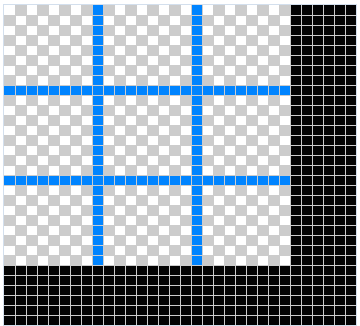Every cell should be 8x8 px with two vertical and two horizontal borders in total. => 26 px x 26 px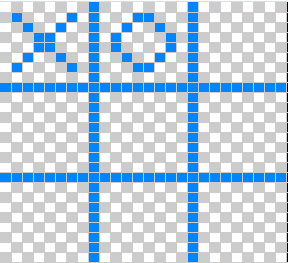The pixel maps are fixed templates for every cell.

### Draw the grid

``````import numpy as np
from skimage.draw import line
from skimage import io
from matplotlib import pyplot as plt

def get_grid():
img = np.zeros((26, 26), dtype=np.uint8)
rr, cc = line(8, 0, 8, 25)
img[rr, cc] = 1
rr, cc = line(17, 0, 17, 25)
img[rr, cc] = 1
rr, cc = line(0, 8, 25, 8)
img[rr, cc] = 1
rr, cc = line(0, 17, 25, 17)
img[rr, cc] = 1
return img

io.imshow(get_grid())
plt.show()
``````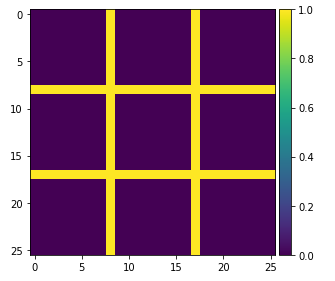### Draw circles and Xses

``````def draw_x(img, offset_x=0, offset_y=0):
rr, cc = line(1+offset_y, 1+offset_x, 6+offset_y, 6+offset_x)
img[rr, cc] = 1
rr, cc = line(6+offset_y, 1+offset_x, 1+offset_y, 6+offset_x)
img[rr, cc] = 1
return img

def draw_o(img, offset_x=0, offset_y=0):
rr, cc = circle_perimeter(3+offset_y, 3+offset_x, 2)
img[rr, cc] = 1
return img

img = get_grid()
img = draw_x(img, offset_x=9, offset_y=0)
img = draw_o(img)
img = draw_o(img, offset_x=18, offset_y=0)

io.imshow(img)
plt.show()
``````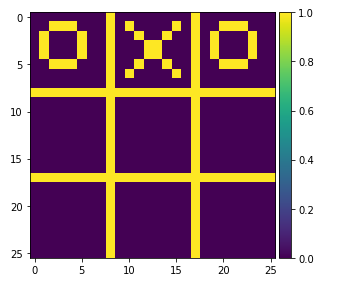### Draw a full game state

``````def draw_num_state(num_state):
img = get_grid()
for y in range(0, 3):
y_offset = y * 9
for x in range(0, 3):
x_offset = x * 9
i = y * 3 + x
cell_type = num_state[i]
if cell_type == 1:
draw_x(img, offset_x=x_offset, offset_y=y_offset)
elif cell_type == 2:
draw_o(img, offset_x=x_offset, offset_y=y_offset)
return img

test_num_state = [1, 2, 1, 2, 1, 2, 1, 0, 0]
img = draw_num_state(test_num_state)

io.imshow(img)
plt.show()
``````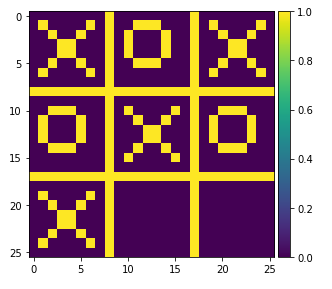### Generate samples

The following code generates multiple game states and images of them. They are not valid though.

``````def generate_game_states(n=10):
imgs = []
states = []
for i in range(n):
states.append(np.random.choice(3, 9))
imgs.append(draw_num_state(states[-1]))
return imgs, states

def draw_game_states(images, num_states):
for row in zip(images, num_states):
print("GAME STATE: {}".format(row))
io.imshow(row)
plt.show()

images, num_states = generate_game_states()
draw_game_states(images, num_states)
``````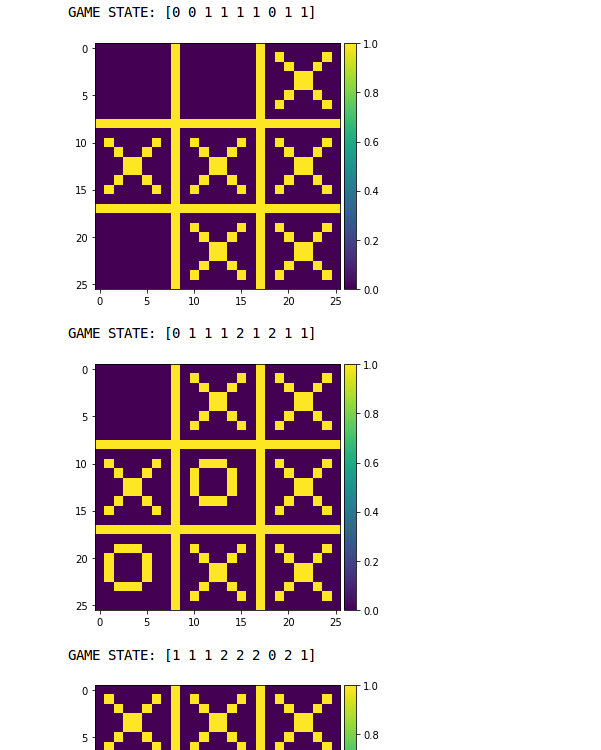## CNN

Now that we can generate data we want to train a model. Training one single CNN would result in 19.683 states (all possible combinations which is 3^9). That is why I want to train a classifier for each cell.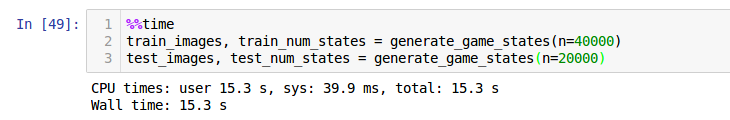``````%%time

train_images, train_num_states = generate_game_states(n=5000)
train_images = np.array(train_images)
train_num_states = np.array(train_num_states)

test_images, test_num_states = generate_game_states(n=3000)
test_images = np.array(test_images)
test_num_states = np.array(test_num_states)
``````

### Classifying one cell

The following CNN shall only be trained on the three labels “Empty”, “X” or “O”.

``````
import tensorflow as tf

# tf.logging.set_verbosity(tf.logging.INFO)

def cnn_model_fn(features, labels, mode):
# Input Layer
# Reshape X to 4-D tensor: [batch_size, width, height, channels]
# The images are 26x26 pixels, and have one color channel
input_layer = tf.reshape(features["x"], [-1, 26, 26, 1])

# Convolutional Layer #1
# Computes 32 features using a 5x5 filter with ReLU activation.
# Input Tensor Shape: [batch_size, 26, 26, 1]
# Output Tensor Shape: [batch_size, 26, 26, 32]
conv1 = tf.layers.conv2d(
inputs=input_layer,
filters=32,
kernel_size=[5, 5],
activation=tf.nn.relu)

# Pooling Layer #1
# First max pooling layer with a 2x2 filter and stride of 2
# Input Tensor Shape: [batch_size, 26, 26, 32]
# Output Tensor Shape: [batch_size, 13, 13, 32]
pool1 = tf.layers.max_pooling2d(inputs=conv1, pool_size=[2, 2], strides=2)

# Convolutional Layer #2
# Computes 64 features using a 5x5 filter.
# Input Tensor Shape: [batch_size, 13, 13, 32]
# Output Tensor Shape: [batch_size, 13, 13, 64]
conv2 = tf.layers.conv2d(
inputs=pool1,
filters=64,
kernel_size=[5, 5],
activation=tf.nn.relu)

# Pooling Layer #2
# Second max pooling layer with a 2x2 filter and stride of 2
# Input Tensor Shape: [batch_size, 13, 13, 64]
# padding same => 13x13 -> 14x14
# Output Tensor Shape: [batch_size, 7, 7, 64]
pool2 = tf.layers.max_pooling2d(inputs=conv2, pool_size=[2, 2], strides=2, padding='SAME')

# Flatten tensor into a batch of vectors
# Input Tensor Shape: [batch_size, 7, 7, 64]
# Output Tensor Shape: [batch_size, 7 * 7 * 64]
pool2_flat = tf.reshape(pool2, [-1, 7 * 7 * 64])

# Dense Layer
# Densely connected layer with 1024 neurons
# Input Tensor Shape: [batch_size, 7 * 7 * 64]
# Output Tensor Shape: [batch_size, 1024]
dense = tf.layers.dense(inputs=pool2_flat, units=1024, activation=tf.nn.relu)

# Add dropout operation; 0.6 probability that element will be kept
dropout = tf.layers.dropout(
inputs=dense, rate=0.4, training=mode == tf.estimator.ModeKeys.TRAIN)

# Logits layer
# Input Tensor Shape: [batch_size, 1024]
# Output Tensor Shape: [batch_size, 3]
logits = tf.layers.dense(inputs=dropout, units=3)

predictions = {
# Generate predictions (for PREDICT and EVAL mode)
"classes": tf.argmax(input=logits, axis=1),
# Add `softmax_tensor` to the graph. It is used for PREDICT and by the
# `logging_hook`.
"probabilities": tf.nn.softmax(logits, name="softmax_tensor")
}
if mode == tf.estimator.ModeKeys.PREDICT:
return tf.estimator.EstimatorSpec(mode=mode, predictions=predictions)

# Calculate Loss (for both TRAIN and EVAL modes)
loss = tf.losses.sparse_softmax_cross_entropy(labels=labels, logits=logits)

# Configure the Training Op (for TRAIN mode)
if mode == tf.estimator.ModeKeys.TRAIN:
train_op = optimizer.minimize(
loss=loss,
global_step=tf.train.get_global_step())
return tf.estimator.EstimatorSpec(mode=mode, loss=loss, train_op=train_op)

# Add evaluation metrics (for EVAL mode)
eval_metric_ops = {
"accuracy": tf.metrics.accuracy(
labels=labels, predictions=predictions["classes"])}
return tf.estimator.EstimatorSpec(
mode=mode, loss=loss, eval_metric_ops=eval_metric_ops)

def train(train_data, train_labels, test_data, test_labels, cell_index=0, training_steps=1000):
# Create the Estimator
classifier = tf.estimator.Estimator(
model_fn=cnn_model_fn, model_dir="./ttt_convnet_model_{}".format(cell_index))

# Set up logging for predictions
# Log the values in the "Softmax" tensor with label "probabilities"
tensors_to_log = {"probabilities": "softmax_tensor"}
logging_hook = tf.train.LoggingTensorHook(
tensors=tensors_to_log, every_n_iter=500)

# Train the model
train_input_fn = tf.estimator.inputs.numpy_input_fn(
x={"x": train_data},
y=train_labels,
batch_size=100,
num_epochs=None,
shuffle=True)

classifier.train(
input_fn=train_input_fn,
steps=training_steps, #20000
hooks=[logging_hook])

# Evaluate the model and print results
eval_input_fn = tf.estimator.inputs.numpy_input_fn(
x={"x": test_data},
y=test_labels,
num_epochs=1,
shuffle=False)

eval_results = classifier.evaluate(input_fn=eval_input_fn)
print(eval_results)

``````

This CNN is an overkill for that static data set we have generated. But maybe in the future I am going to add some error to the generator…

Run: `train(train_images, train_num_states[:, 0], test_images, test_num_states[:, 0], cell_index=0)`

The CNN ran 15 minutes. In the end it returned as part of the evaluation on the test dataan accuracy of 1.0.

``````INFO:tensorflow:Saving dict for global step 1202: accuracy = 1.0, global_step = 1202, loss = 0.0017934386
{'loss': 0.0017934386, 'global_step': 1202, 'accuracy': 1.0}
``````

### Classifying all cells

Now I can run this for every cell like this:

``````%%time
for i in range(9):
train(train_images, train_num_states[:, i], test_images, test_num_states[:, i], cell_index=i)
``````

“CPU times: user 1h 41min 26s, sys: 9min 48s, total: 1h 51min 15s Wall time: 36min 40s”

And to make a full prediction I will have to load all models into different graphs and collect their predictions:

``````tf.logging.set_verbosity(tf.logging.ERROR)

img = get_grid()
prediction = []
for i in range(9):
with tf.Session() as sess:
graph_path = './ttt_convnet_model_{}/model.ckpt-1000.meta'.format(i)
new_saver = tf.train.import_meta_graph(graph_path)
new_saver.restore(sess, tf.train.latest_checkpoint('./ttt_convnet_model_{}'.format(i)))
classifier = tf.estimator.Estimator(model_fn=cnn_model_fn, model_dir="./ttt_convnet_model_{}".format(i))
predict_input_fn = tf.estimator.inputs.numpy_input_fn(
x={"x": np.array([img])},
num_epochs=1,
shuffle=False
)
classification = next(classifier.predict(predict_input_fn))
prediction.append(classification['classes'])

prediction
``````

Argh! This takes 11.2 seconds. I guess it’s the worst way to do it but works for now…

Okay now we make a test run:

``````
def test_predict():
train_images, train_num_states = generate_game_states(n=1)
train_images = np.array(train_images)
train_num_states = np.array(train_num_states)

draw_game_states(train_images, train_num_states)

print("predict...")
print(predict(train_images))

``````

And voilà - it works! :)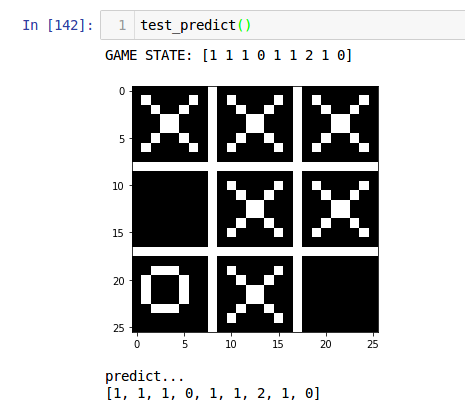## How to continue

The following ideas would be fun starting points for new experiments:

• Create more realistic pixel maps with variation (or even a data set)
• Detect whether a state is legal or not
• Check if there is already a winner
• Build a KI for tic tac toe without telling it the rules